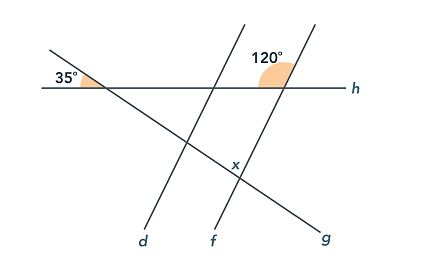# In the figure below, lines d and f are parallel and the angle measures are as given. What is the value of x?85

Explanation

Vertical angles are the angles opposite of each other that are created when two lines cross. Vertical angles are always equal. So, the angle vertical to the 35-degree angle also has a measure of 35 degrees. The supplement of the 120-degree angle has a measure of 60 degrees (all straight lines measure 180 degrees), so we then have a triangle with angles measuring 35, 60, and x degrees, as shown in the figure provided. Since the angles of a triangle add to 180 degrees:

35 + 60 + x = 180
x = 180 − 35 − 60
x = 85 degrees